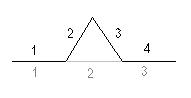ABARIM
Publications
Koch's snowflake or triangle: proof of infinity around limit

# Koch's snowflake or Koch's triangle

## Helge von Koch's Snowflake or Triangle

In 1904 the Swedish mathematician Helge von Koch created a work of art that became known as Koch's Snowflake or Koch's Triangle. It's formed from a base or parent triangle, from which sides grow smaller triangles, and so ad infinitum. That means that the circumference of Koch's Snowflake or Koch's Triangle keeps getting larger until infinity, while the surface it surrounds stays well within the order of magnitude of the parent triangle.

Fellow mathematicians and philosophers alike objected to such a paradox, but no amount of objections could make it ever go away. The Koch Concoction was there to stay. And proving that indeed the circumference is infinite, while the surface stays finite, isn't a big deal either:

## Proof that the Surface S is finite

 Generation n Surface of one single triangle Amount of triangles at generation n Total surface added at generation n 0 A 1 A 1 1/9 A 3*40 3*40 * 1/9 A 2 1/92 A 3*41 3*41 * 1/92 A 3 1/93 A 3*42 3*42 * 1/93 A 4 1/94 A 3*43 3*43 * 1/94 A n 1/9n A 3*4n-1 3*4n-1 * 1/9n A

## Total surface up to generation n

S=A + 3*40 * 1/91 A + 3*41 * 1/92 A + 3*42 * 1/93 A + ...+ 3*4n-1 * 1/9n A

## Both sides times 4 * 1/9

S * 4/9 = (4/9) * A + 3*41 * 1/92 A + 3*42 * 1/93 A +...+ 3*4n-1 * 1/9n A + 3*4n * 1/9n+1 A

## Subtracting S- 4/9 S to ditch the infinite tail

S- 4/9 S = A + 3*40/91 A - 4/9 A - 3*4n/9n+1 A

5/9 S = 5/9 A + 3/9 A - 3*4n/9n+1 A = 8/9 A - 3*4n/9n+1 A

S = 8/5 A - 9/5 * 3 * 4n/9n+1 = 8/5 A - 3/5 * (4/9)n

##### Conclusion:

The final surface of the Koch Snow Flake will be 1 3/5 times the surface of the parent triangle after an infinite number of generations.

## Proof that the circumference L is infiniteEvery generation will cause an increase in length of the curve of 4/3 times a segment of the previous generation. Let the length of the side of the parent triangle be R.

L = 3R + 3* 40/31 R + 3*41/32 R+...+ 3*4n-1/3n R
L =3R + (4/3)0 R + (4/3)1 R + (4/3)2 R +...+ (4/3)n-1 R

## Both sides times 4/3

4/3 L = 4R + (4/3)1 R + (4/3)2 R +...+ (4/3)n-1 R + (4/3)n R

## Subtracting 4/3 L - L to ditch the infinite tail

4/3 L - L = 1/3 L = 4R + (4/3)n R - 3R - (4/3)0 R = (4/3)nR

L = 4 * (4/3)n-1

##### Conclusion:

The final length of the Koch Snow Flake will be infinite after an infinite number of generations.